﻿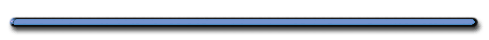Floating Cannon Ball

| Onsite |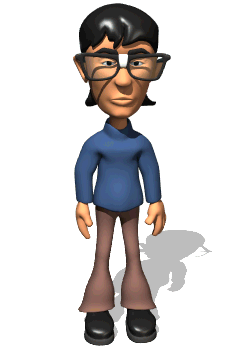"Why can mercury make a cannon ball float?""Mercury must be  more dense than the steel cannonball"

Review

Which force represents the weight force?Weight - net gravitational force on an object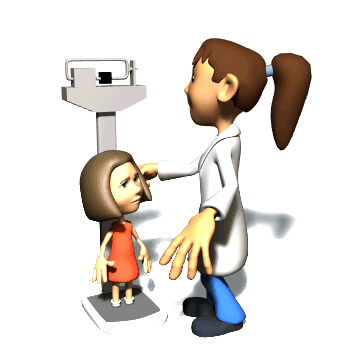w = Fg = mg (N) (kg)(m/s2)

g = acceleration due to gravityEarth: g = 9.8 m/s2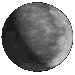Moon: g = 1.6 m/s2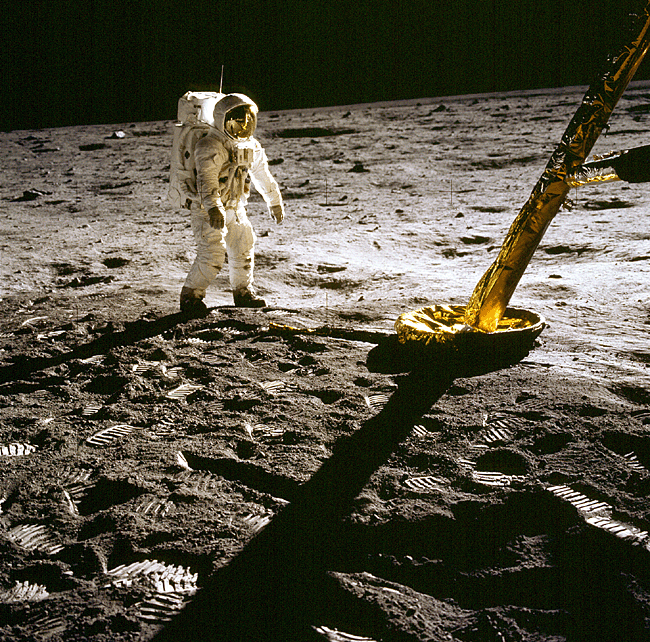Apollo 11 astronaut Edwin Aldrin stands near the leg of the lunar module on the Moon. Despite the fact that the backpacks and astronauts only weighed 1/6 on their 350 pound Earth weight, their center of gravity was shifted so they had to lean slightly forward to balance. Footprints are clearly visible in the foreground.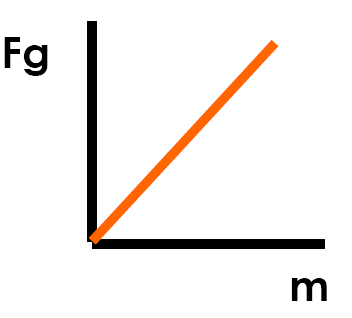slope = g

 g (m/s2) = Fg (N) m (kg)

Mass - amount of matter an object possesses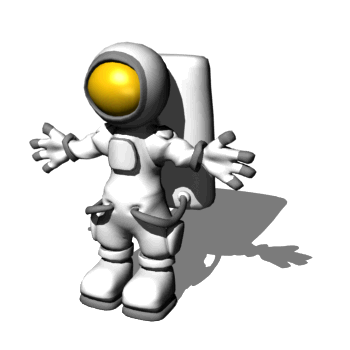Weightless
Not Massless!Did you know that an astronaut can be up to 2 inches taller after returning from space? Why?

The cartilage disks in their spine
expand in the absence of gravity

What is the relationship
between mass and distance?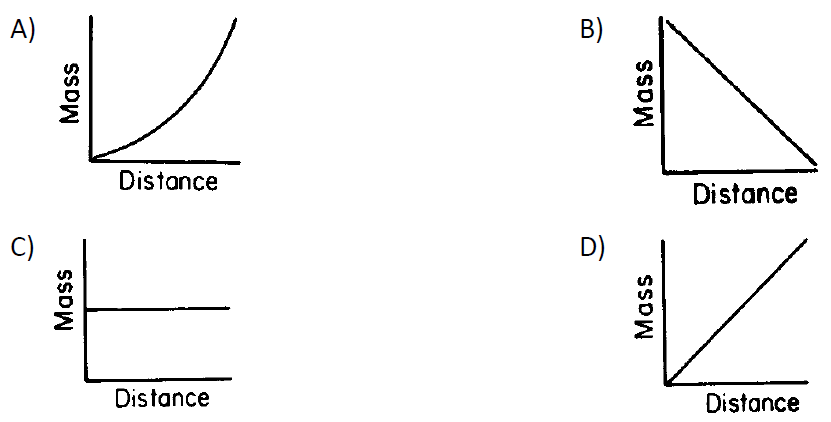Choice 1
Mass is not affected by distance
(only weight)1 kg  ≈ 2.2 lbs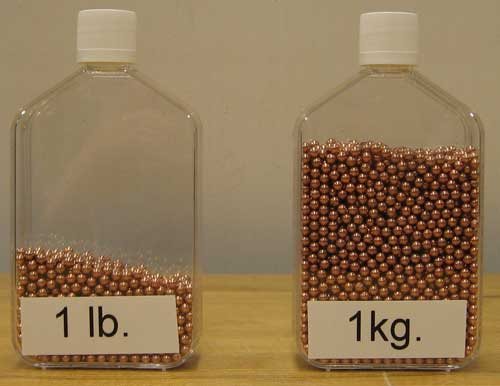1 lbs  ≈ 5 N

Average Senior Weighs

120 lbs 600 N

Finding acceleration
due to gravity using weight

 Ex) A satellite having a mass of 25. kg experiences a gravitational force of 200 N.   Find the acceleration due to gravity at this location.

Ex) A satellite having a mass of 25. kg experiences a gravitational force of 200 N. Find the acceleration due to gravity at this location.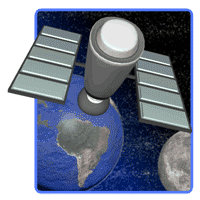g (m/s2) = Fg (N) m (kg)

g = 200 N/25 kg

a  = 8 m/s2

or 8 N/kg

Ex) A rocket weighs 18,000 N at the earth's surface. If the rocket rises to a height where its distance from the earth is 3 earth radii, its weight will be ...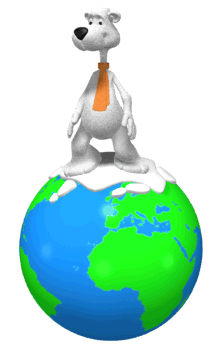When an object is on the earth,
it is one earth radius away.

 F = Gm1m2 r2

Distance from earth to rocket triples so, ....

....weight is 1/9 as great

w = 2000 N

(original weight, 18,000 N)

# Wringing out Water on the ISS - for Science!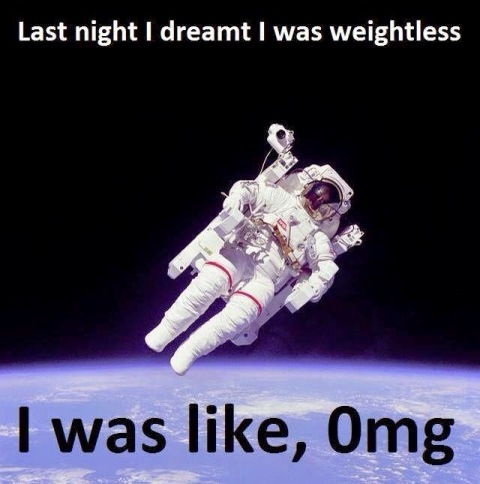Rank the gravitational forces from greatest to smallest.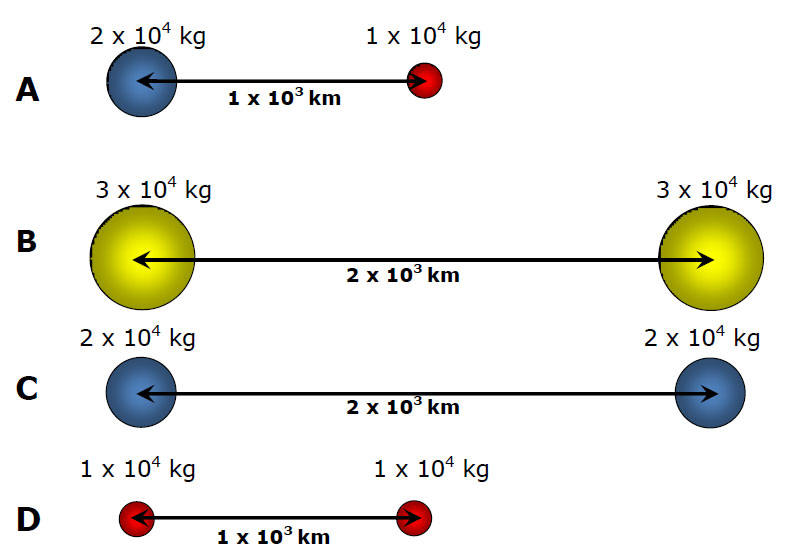Enrichmentwww.wpclipart.comThe Elegant Universe - PBSMythbusters Moon Landing Hoax 4 HQ